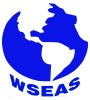J. C. Ndogmo

### Authors and WSEAS

J. C. Ndogmo# WSEAS Transactions on Mathematics

Print ISSN: 1109-2769
E-ISSN: 2224-2880

Volume 17, 2018

Notice: As of 2014 and for the forthcoming years, the publication frequency/periodicity of WSEAS Journals is adapted to the 'continuously updated' model. What this means is that instead of being separated into issues, new papers will be added on a continuous basis, allowing a more regular flow and shorter publication times. The papers will appear in reverse order, therefore the most recent one will be on top.

Volume 17, 2018

Generalization of non-Cartan Symmetries to Arbitrary Dimensions

AUTHORS: J. C. Ndogmo

ABSTRACT: Second order scalar ordinary differential equations (ODEs) which are linearizable possess special types of symmetries. These are the only symmetries which are non fiber-preserving in the linearized form of the equation, and they are called non-Cartan symmetries and known only for scalar ODEs. We give explicit expressions of nonCartan symmetries for systems of ODEs of arbitrary dimensions and show that they form an abelian Lie algebra. It is however shown that the natural extension of these non-Cartan symmetries to arbitrary dimensions is applicable only to the natural extension of scalar second order equations to higher dimensions, that is, to equivalence classes under point transformations of the trivial vector equation. More precisely, it is shown that non-Cartan symmetries characterize linear systems of ODEs reducible by point transformation to their trivial counterpart, and we verify that they do not characterize nonlinear systems of ODEs having this property. It is also shown amongst others that the non-Cartan property of a symmetry vector is coordinate-free. Some examples of application of these results are discussed.

KEYWORDS: Lie point symmetry algebras, non-Cartan symmetries, Systems of ordinary differential equations

REFERENCES:

 G. Chavchanidze, Non-Noether symmetries and their influence on phase space geometry, Journal Of Geometry And Physics, 48, 2003, pp. 190– 202.

 J. Krause and L. Michel, Equations differentielles lin ´ eaires d’ordre ´ n > 2 ayant une algebre de Lie de sym ` etrie de dimension ´ n + 4, C.R. Acad. Sci. Paris 307, 1988, pp. 905–910.

 S. Lie, Klassifikation und integration von gewohnlichen differentialgleichungen zwischen ¨ x, y, die eine gruppe von transformationen gestatten: III. Arch. Mat. Nat. 8, 1883, pp. 371-427. S. Lie, Lies Gessammelte Abhandlundgen 5, 1924, pp. 362–427 paper XIY (reprint).

 A. Gonzalez–L ´ opez, Symmetries of linear sys- ´ tems of second–order ordinary differential equations, J. Math. Phys. 29, 1988, pp. 1097–1105.

 Sergey V. Meleshko, On linearization of thirdorder ordinary differential equations, J. Phys. A: Math. Gen. 39, 2006, pp. 15135–15145.

 S. Moyo and P.G.L. Leach, Exceptional properties of second and third order ordinary differential equations of maximal symmetry, Journal of Mathematical Analysis and Applications 252, 2000, pp. 840–863.

 J.C. Ndogmo, Structure of Lie point and variational symmetry algebras for a class of odes, Commun. Nonlinear Sci. Numer. Simul. 57, 2018, pp. 177–185.

 J.C. Ndogmo and F.M. Mahomed, On certain properties of linear iterative equations, Cent. Eur. J. Math. 12, 2014, pp. 648–657.

 J.C. Ndogmo, Generation and Identification of Ordinary Differential Equations of Maximal Symmetry Algebra, Abstr. Appl. Anal. 2016, Article ID 1796316, 9 pages.

 J.C. Ndogmo, Characterization of canonical classes for systems of linear ODEs, Math Meth Appl Sci 40, 2017, pp. 4928–4936.

 J.C. Ndogmo, Invariants of solvable Lie algebras of dimension six., J. Phys. A: Math. Gen. 33, 2000, 2273–2287.

 P.J. Olver, Equivalence, invariants, and symmetry, Cambridge University Press, Cambridge 1995.

 A.A. Sagle and R.E. Walde Introduction to Lie Groups and Lie Algebras, Academic Press, New York 1973.

WSEAS Transactions on Mathematics, ISSN / E-ISSN: 1109-2769 / 2224-2880, Volume 17, 2018, Art. #2, pp. 6-12